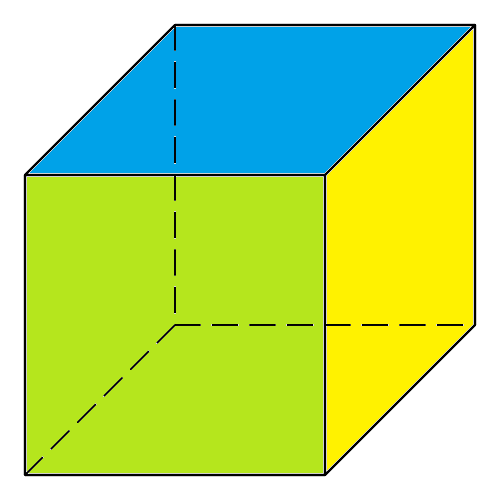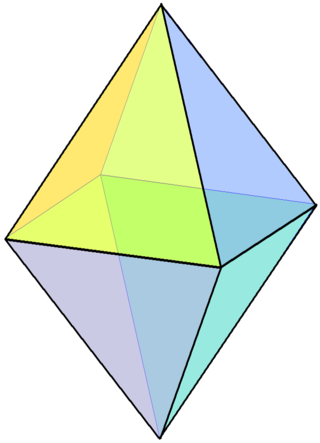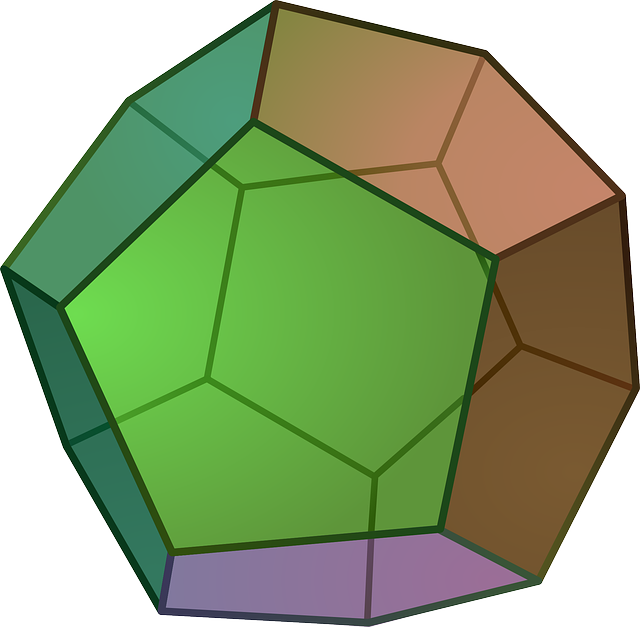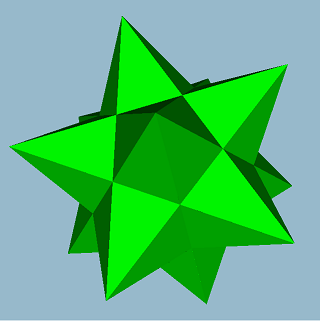# Euler's Formula

Euler's Formula is a relationship between the numbers of faces, edges and vertices (corners) of a convex polyhedron (a 3-D shape with flat faces and straight edges that doesn't have any dents in it).

It states that the number of vertices plus the number of faces minus the number of edges always equals $2$.

We write Euler's Formula as

$V + F - E = 2$
where $V$ is the number of vertices, $F$ is the number of faces and $E$ is the number of edges of the polyhedron.A tetrahedron is a convex polyhedron. It has $4$ vertices, $4$ faces and $6$ edges. Let's test out Euler's Formula:

$V + F - E = 4 + 4 - 6 = 2$

## The Platonic Solids

Now, let's try Euler's formula on the rest of the Platonic solids.

### The Cube (or Hexahedron)The cube has $8$ vertices, $6$ faces and $12$ edges. So,

$V + F - E = 8 + 6 - 12 = 2$

### The OctahedronThe octahedron has $6$ vertices, $8$ faces and $12$ edges. So,

$V + F - E = 6 + 8 - 12 = 2$

### The DodecahedronThe dodecahedron has $20$ vertices, $12$ faces and $30$ edges. So,

$V + F - E = 20 + 12 - 30 = 2$

### The IcosahedronThe icosahedron has $12$ vertices, $20$ faces and $30$ edges. So,

$V + F - E = 12 + 20 - 30 = 2$

## Are There Solids that Euler's Formula Doesn't Work For?

Yes, Euler's formula is only guaranteed to work for convex polyhedrons. Here's a polyhedron that isn't convex. It's called a small stellated dodecahedron:The small stellated dodecahedron (try saying that 10 times quickly) has 12 vertices, 12 faces and 30 edges. So,

$V + F - E = 12 + 12 - 30 = -6$
which is nothing like $2$. So, Euler's theorem doesn't work for this solid. This is because it isn't convex: it has lots of dents.

## Euler Characteristic

Mathematicians love to generalise things. One generalisation of Euler's formula is the Euler Characteristic. The Euler characteristic can take on all sorts of different values (not just 2) and we can find the Euler characteristic of non-convex polyhedra and other solids that are not polyhedra. The Euler characteristic is defined to be

$\chi = V + F - E$

Mathematicians are interested in the Euler characteristic as it doesn't change if you deform a solid: twist or bend it, squeeze it or shrink it. It is what we call a topological invariant. Let's have a look at a few examples of Euler characteristics

Solid Picture $\chi$
Sphere$2$
Klein Bottle$0$
3-holed Torus$-4$
Small Stellated Dodecahedron$-6$

Note: we know that the Euler characteristic of a sphere is $2$ because we can pump up any of the Platonic solids to form a sphere, and their Euler characteristics are all $2$. We say that the Platonic solids are homeomorphic to a sphere because we can deform them (by pumping them up) to form a sphere.

### Description

There are several lessons related to 3D geometry such as

1. Euler's formula
2. Vertices, Edges and Faces
3. Volumes of 3D shapes
4. etc

Even though we've titled this lesson series to be more inclined for Year 10 or higher students, however, these lessons can be read and utilized by lower grades students.

### Prerequisites

Understanding of 3D shapes

### Audience

Year 10 or higher, but suitable for Year 8+ students

### Learning Objectives

Get to know 3D Geometry

Author: Subject Coach
You must be logged in as Student to ask a Question.